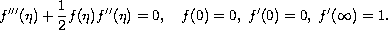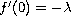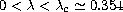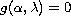Electron. J. Diff. Eqns., Vol. 2007(2007), No. 79, pp. 1-8.

### Degeneracy in the Blasius problem Faiz Ahmad

Abstract:
The Navier-Stokes equations for the boundary layer are transformed, by a similarity transformation, into the ordinary Blasius differential equation which, together with appropriate boundary conditions constitutes the Blasius problem,The well-posedness of the Navier-Stokes equations is an open problem. We solve this problem, in the case of constant flow in a boundary layer, by showing that the Blasius problem is ill-posed. If the second condition is replaced by, then degeneracy occurs for. We investigate the problem analytically to explain this phenomenon. We derive a simple equation, whose roots, for a fixed, determine the solutions of the problem. It is found that the equation has exactly two roots forand no root beyond this point. Since an arbitrarily small perturbation of the boundary condition gives rise to an additional solution, which can be markedly different from the unperturbed solution, the Blasius problem is ill-posed.

Submitted June 27, 2006. Published May 25, 2007.
Math Subject Classifications: 34A12, 34A34, 47J06.
Key Words: Navier-Stokes equations; Blasius problem; degeneracy; Wang equation; well-posed problem; ill-posed problem.

Show me the PDF file (199K), TEX file, and other files for this article.

 Faiz Ahmad Department of Mathematics Faculty of Science, King Abdulaziz University P. O. Box 80203, Jeddah 21589, Saudi Arabia email: faizmath@hotmail.com   Phone:+966 2 2523205